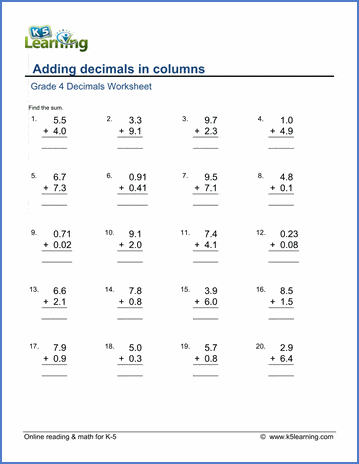## Introductory decimal worksheets

Our grade 4 decimal worksheets provide practice in simple decimal addition and subtraction.  These combined with our conversion of simple fractions to and from decimals worksheets provide an introduction to math with decimals.

## Comparing and ordering decimals

Example

Compare decimals (1-2 digits)   7.89 < 12.4
Order decimals (1-2 digits)   0.4, 0.96, 3.1, 16.8

4.5 + 1.5
1 digit plus 2 digit   4.5 + 1.55 =
1 or 2 digits   4.55 + 1.55 =

1.23

+ 2.34

## Decimal Subtraction

Decimal subtraction (1 digit)
3.6 - 1.3 =
Decimal subtraction - 1 or 2 digits   3.65 - 1.36 =
Decimal subtraction  (missing number)   ___ - 1.5 = 0.1
Decimal subtraction in columns

3.57

- 2.34

## Money notation

Decimals and money notation   \$3.57
- 2.34

## Decimal word problems

Adding and subtracting decimals word problems   Word problems